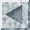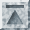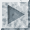# Regression Analysis

The most commonly performed statistical procedure in SST is multiple regression analysis. The `REG` command provides a simple yet flexible way compute ordinary least squares regression estimates. Options to the `REG` command permit the computation of regression diagnostics and two-stage least squares (instrumental variables) estimates.

## The linear regression model

In the linear regression model, the dependent variable is assumed to be a linear function of one or more independent variables plus an error introduced to account for all other factors:
In the above regression equation, y_i is the dependent variable, x_i1, ...., x_iK are the independent or explanatory variables, and u_i is the disturbance or error term. The goal of regression analysis is to obtain estimates of the unknown parameters Beta_1, ..., Beta_K which indicate how a change in one of the independent variables affects the values taken by the dependent variable.

Applications of regression analysis exist in almost every field. In economics, the dependent variable might be a family's consumption expenditure and the independent variables might be the family's income, number of children in the family, and other factors that would affect the family's consumption patterns. In political science, the dependent variable might be a state's level of welfare spending and the independent variables measures of public opinion and institutional variables that would cause the state to have higher or lower levels of welfare spending. In sociology, the dependent variable might be a measure of the social status of various occupations and the independent variables characteristics of the occupations (pay, qualifications, etc.). In psychology, the dependent variable might be individual's racial tolerance as measured on a standard scale and with indicators of social background as independent variables. In education, the dependent variable might be a student's score on an achievment test and the independent variables characteristics of the student's family, teachers, or school.

The common aspect of the applications described above is that the dependent variable is a quantitative measure of some condition or behavior. When the dependent variable is qualitative or categorical, then other methods (such as logit or probit analysis, described in Chapter 7) might be more appropriate.

## Ordinary least squares estimation

The usual method of estimation for the regression model is ordinary least squares (OLS). Let b_1, ..., b_K denote the OLS estimates of Beta_1, ..., Beta_K. The predicted value of y_i is:
The error in the OLS prediction of y_i, called the residual, is:
The basic idea of ordinary least squares estimation is to choose estimates Beta_1, ..., Beta_K to minimize the sum of squared residuals:It can be shown that:
where X is an n * k matrix with (i,k)th element x_ki, y is an n * k vector with typical element y_i, and b is a k * 1 vector with typical element b_k.

## Assumptions for regression analysis

The least squares fitting procedure described below can be used for data analysis as a purely descriptive technique. However, the procedure has strong theoretical justification if a few assumptions are made about how the data are generated. The starting point is the regression equation presented above which describes some causal or behavioral process. The independent variables play the role of experimental or treatment variables, though in few social science applications will the investigator actually have control over the values of the independent variables. The error term captures the effects of all omitted variables. In an experiment, randomization of the treatments (independent variables) ensures that the omitted factors (the disturbances) are uncorrelated with the treatments. This greatly simplifies inference. Non-experimental researchers, however, must substitute assumptions for experimental controls. The validity of non-experimental results therefore depends critically upon the accuracy of the assumptions. We present one set of assumptions, known as the Gauss-Markov assumptions, that are sufficient to guarantee that ordinary regression estimates will have good properties.

First, we assume that the errors u_i have an expected value of zero: E(u_i ) = 0 This means that on average the errors balance out.

Second, we assume that the independent variables are non-random. In an experiment, the values of the independent variable would be fixed by the experimenter and repeated samples could be drawn with the independent variables fixed at the same values in each sample. As a consequence of this assumption, the indenpendent variables will in fact be independent of the disturbance. For non-experimental work, this will need to be assumed directly along with the assumption that the independent variables have finite variances.

Third, we assume that the independent variables are linearly independent. That is, no independent variable can be expressed as a (non-zero) linear combination of the remaining independent variables. The failure of this assumption, known as multicollinearity, clearly makes it infeasible to disentangle the effects of the supposedly independent variables. If the independent variables are linearly dependent, SST will produce an error message (`singularity in independent variables`) and abort the `REG` command.

Fourth, we assume that the disturbances u_i are homoscedastic:
This means that the variance of the disturbance is the same for each observation.

Fifth, we assume that the disturbances are not autocorrelated:
This means disturbances associated with different observations are uncorrelated.

## Properties of the OLS estimator

If the first three assumptions above are satisfied, then the ordinary least squares estimator b will be unbiased: E(b) = beta Unbiasedness means that if we draw many different samples, the average value of the OLS estimator based on each sample will be the true parameter value beta. Usually, however, we have only one sample, so the variance of the sampling distribution of b is an important indicator of the quality of estimates obtained.

If all five of the assumptions above hold, then it can be shown that the variance of the OLS estimator is given by:
If the independent variables are highly intercorrelated, then the matrix X' X will be nearly singular and the element of (X' X)^-1 will be large, indicating that the estimates of beta may be imprecise.

To estimate Var(b) we require an estimator of sigma^2. It can be shown that:is an unbiased estimator of sigma^2. The square root of (sigma hat)^2 is called the standard error of the regression. It is just the standard deviation of the residuals e_i.

There are two important theorems about the properties of the OLS estimators. The Gauss-Markov theorem states that under the five assumptions above, the OLS estimator b is best linear unbiased. That is, the OLS estimator has smaller variance than any other linear unbiased estimator. (One covariance matrix is said to be larger than another if their difference is positive semi-definite.) If we add the assumption that the disturbances u_i have a joint normal distribution, then the OLS estimator has minimum variance among all unbiased estimators (not just linear unbiased estimators).

Although the preceding theorems provide strong justification for using the OLS estimator, it should be realized that OLS is rather sensitive to departures from the assumptions. A few outliers (stray observations generated by a different process) can strongly influence the OLS estimates. SST provides useful diagnostic tools for detecting data problems that we discuss below.

## Use of the REG command

To estimate a regression in SST, you need to specify one or more dependent variables (in the `DEP` subop) and one or more independent variables (in the `IND` subop). Unlike some other programs, SST does not automatically add a constant to your independent variables. If you want one, you should create a constant and add it to the list of your independent variables. For example, to regress the variable `y` on `x` with an intercept:

```set one=1
reg dep[y] ind[one x]
```

SST will produce two coefficients: an intercept and a slope parameter. The corresponding regression line passes through the point (0,b_0) and has slope equal to b_1:where b_0 is the coefficient of `one` and b_1 is the coefficient of the variable `x`. If, on the other hand, you had omitted the variable `one` from the `IND` subop:

```reg dep[y] ind[x]
```

SST would produce a "regression through the origin". That is, the regression line would pass through the point (0,0) with slope equal to the coefficient of x (b):
For most purposes you will want to include a constant, but SST allows you the flexibility to decide otherwise.

The `IF` and `OBS` subops can be used to restrict the range of observations used in the regression. Only the subset of observations activated by the current `RANGE` statement that meet the criteria set in the `IF` and `OBS` subops will be used. If any of the variables specified in the `IND` or `DEP` subops have missing data for an observation, the entire observation is deleted from the estimation range for that regression. For example, to run a regression of `y` on `x` (and a constant) including only observations one through ten:

```reg dep[y] ind[one x] obs[1-10]
```

Using the `OBS` subop does not affect the observation range for subsequent commands.

SST also allows you to specify multiple dependent variables in the `DEP` subop. If one variable is specified in the `DEP` subop, it will be regressed on the variables specified in the `IND` subop. If more than one variable is specified in the `DEP` subop, separate regressions will be run for each of these variables on the variables listed in the `IND` subop. The same observation range will be used for all regressions.

## An example

We illustrate use of the `REG` command using an example taken from David A. Belsey, Edwin Kuh, and Roy E. Welsch, Regression Diagnostics (Wiley, 1980). The variables in the data set are macroeconomic and demographic indicators for fifty countries for the decade of the 1960's and are used to test a simply life cycle savings model.

A text file containing the data is supplied with your SST program disk (bkw.dat). The following variables are in the file:

```SR       Personal savings rate
POP15    Percentage of population under age 15
POP75    Percentage of population over age 75
PDI      Personal disposable income per capita (constant dollars)
DELPDI   Percentage growth rate of PDI from 1960 to 1970
```

According to the life cycle savings model, savings rates will be highest among middle-aged individuals. Younger individuals, anticipating higher incomes as they become older, will have low savings rates. On the other hand, older individuals will tend to consume whatever savings they accumulated during middle age. The following regression equation is proposed to test this theory:
To replicate the Belsey, Kuh, and Welsch analysis, first we `READ` the data file `bkw.dat` as described in Chapter 2. Then we `LABEL` it, `SET` a variable `one` equal to a vector of ones, and `SAVE` the entire data set. The commands are the following:

```range obs[1-100]
read to[sr pop15 pop75 dpi deldpi] file[bkw.dat]
label var[sr] lab[average personal savings rate]
label var[pop15] lab[percentage population under 15]
label var[pop75] lab[percentage population over 75]
label var[dpi] lab[real disposable income per capita]
label var[deldpi] lab[real disposable income growth rate]
save file[bkw]
set one=1
```

Now we are ready to regress `sr` on `one`, `pop15`, `pop75`, `dpi`, and `deldpi`:

```reg dep[sr] ind[one pop15 pop75 dpi deldpi]
```

The output produced is:

```********** ORDINARY LEAST SQUARES **********
Dependent Variable:        sr

Independent            Estimated            Standard              t-
Variable             Coefficient            Error            Statistic

one              28.5662941          7.3544917          3.8841969
pop15              -0.4611972          0.1446415         -3.1885533
pop75              -1.6914257          1.0835935         -1.5609412
dpi              -0.0003371          0.0009311         -0.3620211
deldpi               0.4096868          0.1961958          2.0881534

Number of Observations                50
R-squared                              0.338458
Corrected R-squared                    0.279655
Sum of Squared Residuals              6.51e+002
Standard Error of the Regression      3.8026627
Mean of Dependent Variable            9.6710000
```

The OLS estimates provide some support for the life cycle model. The t-statistic for the coefficient of `pop15` is -3.18, which would enable us to reject the null hypothesis beta_2 = 0 at conventional significance levels. The coefficient of `pop75`, however, does not achieve significance at 0.05 level. Notice, also, that the income effect is small (a thousand dollars of income is associated with only a 0.3 percent rise in the savings rate) and statistically insignificant, while the income change variable has a positive and statistically significant impact on the savings rate.

## Regression diagnostics

The `REG` procedure also allows you to produce diagnostic statistics to evaluate the regression estimates including:

• predicted values (`PRED`)
• residuals (`RSD`)
• studentized residuals (`SRSD`)
• diagonal elements of the "hat" matrix (`HAT`)
• estimated coefficients (`COEF`)
• covariance matrix (`COVMAT`)

Most of these will be familiar, but we discuss in some detail some of the less well known diagnostics: studentized residuals and the hat matrix. These two diagnostics are discussed in detail in Regression Diagnostics.

## Studentized residuals and the hat matrix

Studentized residuals are helpful in identify outliers which do not appear to be consistent with the rest of the data. The hat matrix is used to identify "high leverage" points which are outliers among the independent variables. The two concepts are related. In the case of studentized residuals, large deviations from the regression line are identified. Since the residuals from a regression will generally not be independently or identically distributed (even if the disturbances in the regression model are), it is advisable to weight the residuals by their standard deviations (this is what is meant by studentization). A similar idea motivates the calculation of the hat matrix (see Regression Diagnostics, p. 17).

The hat matrix H is given by: H = X(X' X)^-1 X' Note that since: b = (X' X)^-1 X' y and by definition: y hat = Xb it follows that: y hat = Hy Since the hat matrix is of dimension n * n, the number of elements in it can become quite large. Usually it suffices to work with only the diagonal elements h_1, ..., h_n:
where x_i is the ith row of the matrix X. Note that:
so that:
since I-H is idempotent. It follows therefore:
so that the diagonal elements of the hat matrix are closely related to the variances of the residuals. To compute the studentized residuals, we divide e_i by an estimate of its variance. Rather than using
, we recompute the regression deleting the ith observation. Denote the corresponding estimate of sigma^2 with the ith observation deleted by s^2 (i) and the corresponding diagonal element of the hat matrix from the regression with the ith observation deleted by h_i tilde. The formula for the studentized residual for the ith observation is:
## Use of the hat matrix diagonal elements

Since y hat = Hy, the diagonal elements of H, the h_i, indicate the effect of a given observation. There are a few useful facts about the diagonal elements of the hat matrix:
where K is the number of independent variables, including the constant if there is one. Belsley, Kuh, and Welch suggest 2p/n as a rough cutoff for determing high leverage points, terming the ith observation a leverage point when h_i exceeds 2p/n.

## Use of studentized residuals

Belsley, Kuh, and Welch point out that the studentized residuals have an approximate t-distribution with n-p-1 degrees of freedom. This means we can assess the significance of any single studentized residual using a t-table (or, equivalently, a table of the standard normal distribution if n is moderately large). For example, we might rerun the above regression and save the studentized residuals by specifying a variable name in the `SRSD` subop:

```reg dep[sr] ind[one pop15 pop75 dpi deldpi] srsd[studrsd]
```

Next, we might investigate which observations have large studentized residuals:

```print var[studrsd] if[abs(studrsd) > 1.96]

OBS       VARIABLES
studrsd
7:         -2.3134
46:          2.8536
```

Next, we could rerun the regression omitting those observations with large studentized residuals:

```reg dep[sr] ind[one pop15 pop75 dpi deldpi] if[abs(studrsd) <= 1.96]

********** ORDINARY LEAST SQUARES **********
Dependent Variable:        sr

Independent            Estimated            Standard              t-
Variable             Coefficient            Error            Statistic

one              28.8187711          6.5324171          4.4116551
pop15              -0.4683572          0.1280318         -3.6581323
pop75              -1.5778925          0.9686178         -1.6290146
dpi              -0.0003989          0.0008229         -0.4846829
deldpi               0.3480148          0.1740605          1.9993897

Number of Observations                48
R-squared                              0.410031
Corrected R-squared                    0.355150
Sum of Squared Residuals              4.85e+002
Standard Error of the Regression      3.3589505
Mean of Dependent Variable            9.6747917
```

In this case the coefficient estimates seem relatively stable with the outliers removed.

## Instrumental variables estimation

SST also allows easy estimation of simulataneous equations models using two stage least squares. Models where some of the independent variables are correlated with the disturbances will be inconsistently estimated by OLS. If, however, a set of instrumental variables (which are correlated with the independent variables, but uncorrelated with the disturbances) is available, it is possible to "purge" the independent variables of their correlation with the disturbance. The instrumental variables are specified in the `IV` subop. Variables in the `IV` subop can overlap with those in the `IND` subop if their are included exogenous variables in the equation. The number of instrumental variables (including included exogenous variables) must be at least as large as the number of independent variables (or else the order condition for identification will not be met).

A simple example of simultaneous equations estimation occurs in estimating a market supply or demand equation. Consider, for example, the supply function for an agricultural commodity. Let `price` be the market price of the commodity in each period and `quantity` the quantity supplied of the commodity. In equilibrium, quantity demanded and quantity supplied are equal. Moreover, `price` and `quantity` are simultaneously determined in the market, so it does not make sense to regress `quantity` on `price` to obtain an estimate of the price elasticity of supply. Suppose, we believe that the supply function also depends on the weather (measured, say, by `rainfall`) while the demand function also depends on population (`populat`) and aggregate personal disposable income (`pdi`), but that these variables are exogenous to the market for this particular commodity. To estimate the supply equation by two stage least squares, give the command:

```reg dep[quantity] ind[one price weather] iv[one weather populat pdi]
```

Remember to include the constant `one` in the `IV` subop, since it is certainly exogenous. For details of simultaneous equations estimation, consult any econometrics text, e.g. H. Theil, Principles of Econometrics (Wiley, 1971), chapters 9-10.